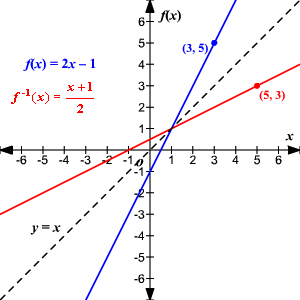# Inverse Functions

The functions $f$ and $g$ are inverse functions if $f\left(g\left(x\right)\right)=x$ for all $x$ in the domain of $g$ and $g\left(f\left(x\right)\right)=x$ for all $x$ in the domain of $f$ .

The inverse of a function $f$ is usually denoted ${f}^{-1}$  and read “ $f$ inverse.”  (Note that the superscript $-1$ in ${f}^{-1}$  is not a exponent).

Suppose that two functions are inverses.  If $\left(a,b\right)$ is a point on the graph of the original function, then the point $\left(b,a\right)$ must be a point on the graph of the inverse function.  The graphs are mirror images of each other with respect to the line $y=x$ .To find the inverse of a function algebraically, interchange the $x$ and $y$ and solve for $y$ .

Example:

Let $h\left(x\right)={x}^{3}+4$

Replace $h\left(x\right)$ with $y$ and interchange $x$ and $y$

$\begin{array}{l}y={x}^{3}+4\\ x={y}^{3}+4\end{array}$

Solve for $y$ : $x-4={y}^{3}$

$\sqrt{x-4}=y={h}^{-1}\left(x\right)$